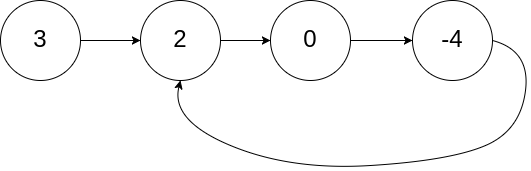# Leetcode 142 - Linked list cycle II

Note:

• Double pointers:
• Just like Linked list cycle I, we should use double pointers but it’s more complicated.
•• By using 2-pointer, we can find the node where two pointers meet.
• Here comes the deduction:
• Assume fast pointer has travelled n rounds inside the cycle.
• Because fast always travels 2 times more than slow, we have 2*(a + b) = a + n*(b + c) + b, which is a = c + (n-1)*b.
• If n = 1, we have a = c.
• Because right now slow is at b, we can use another pointer at the start. And if they both start moving, they will meet at the intersection!.
• Use Set to tell if we’ve iterated the current node before.

Question:

Given the head of a linked list, return the node where the cycle begins. If there is no cycle, return null.

There is a cycle in a linked list if there is some node in the list that can be reached again by continuously following the next pointer. Internally, pos is used to denote the index of the node that tail’s next pointer is connected to (0-indexed). It is -1 if there is no cycle. Note that pos is not passed as a parameter.

Do not modify the linked list.

Example:Code:

Double pointers

Set